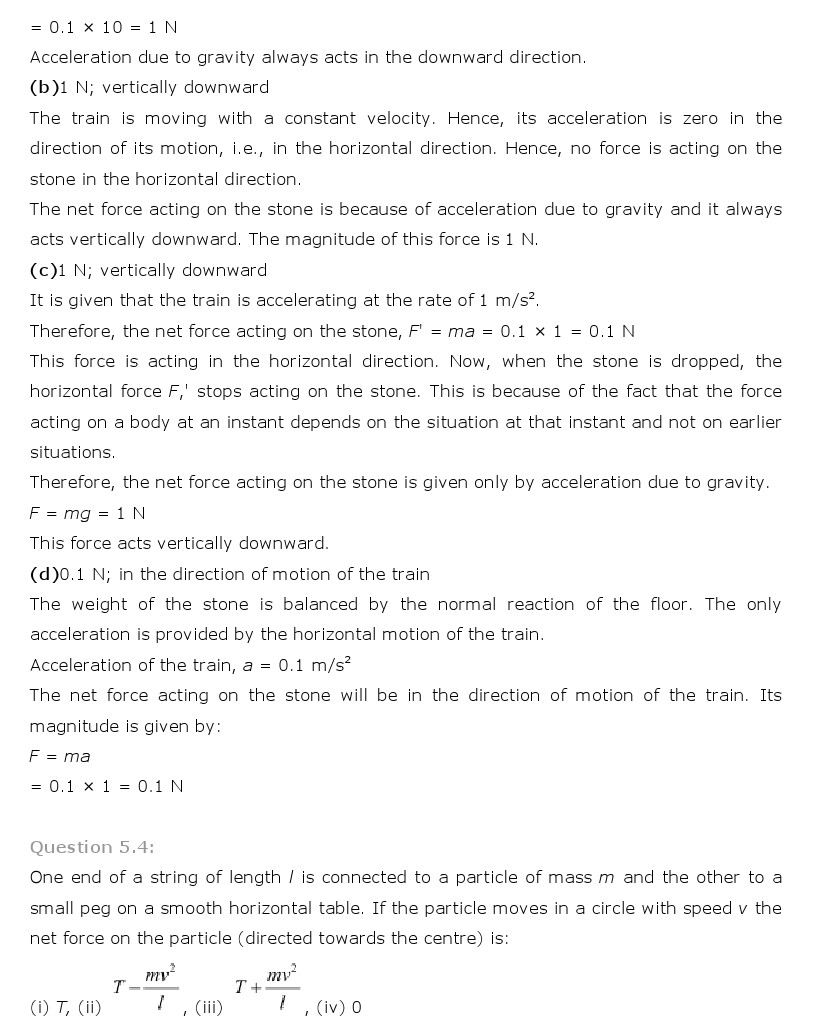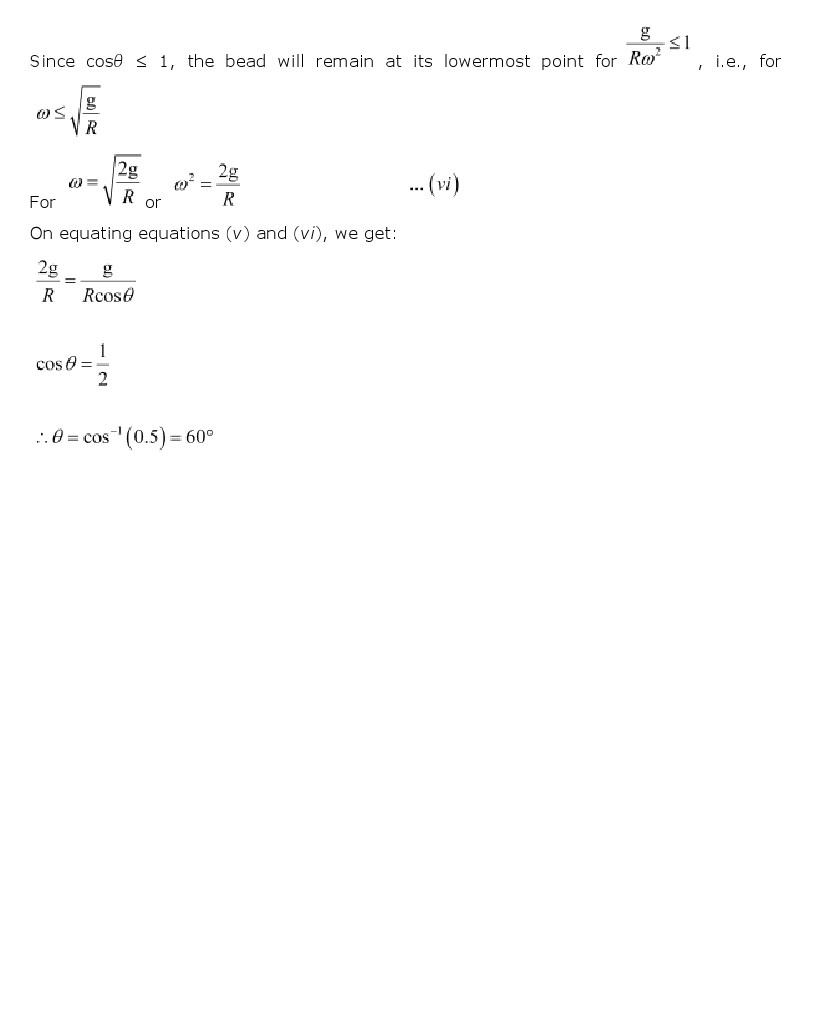# NCERT Solutions Class 11th Physics Chapter 5 Laws of motion

NCERT Solutions Class 11th Physics Chapter 5 Laws of motion

## NCERT Solutions Class 11th Physics Chapter 5 Laws of motion

National Council of Educational Research and Training (NCERT) Book Solutions class 11th
Subject: Physics
Chapter: Chapter 5 Laws of motion

Class 11th Physics Chapter 5 Laws of motion NCERT Solution is given below.CBSE sample papers http://www.cbsesamplepapers.info/
RD sharma solutions for class 11: http://www.cbselabs.com/forums/rd-sharma-class-11-solutions.46/

NCERT Solutions for maths in Video https://www.youtube.com/user/cbsepapers/videos
Newton’s First Law of Motion:
I. Every object in a state of uniform motion tends to remain in that state of motion unless an external force is applied to it.
Newton’s Second Law of Motion:
II. The relationship between an object’s mass m, its acceleration a, and the applied force F is F = ma. Acceleration and force are vectors (as indicated by their symbols being displayed in slant bold font); in this law the direction of the force vector is the same as the direction of the acceleration vector.
This is the most powerful of Newton’s three Laws, because it allows quantitative calculations of dynamics: how do velocities change when forces are applied. Notice the fundamental difference between Newton’s 2nd Law and the dynamics of Aristotle: according to Newton, a force causes only a change in velocity (an acceleration); it does not maintain the velocity as Aristotle held.

This is sometimes summarized by saying that under Newton, F = ma, but under Aristotle F = mv, where v is the velocity. Thus, according to Aristotle there is only a velocity if there is a force, but according to Newton an object with a certain velocity maintains that velocity unless a force acts on it to cause an acceleration (that is, a change in the velocity). As we have noted earlier in conjunction with the discussion of Galileo, Aristotle’s view seems to be more in accord with common sense, but that is because of a failure to appreciate the role played by frictional forces. Once account is taken of all forces acting in a given situation it is the dynamics of Galileo and Newton, not of Aristotle, that are found to be in accord with the observations.
Newton’s Third Law of Motion:
III. For every action there is an equal and opposite reaction.
This law is exemplified by what happens if we step off a boat onto the bank of a lake: as we move in the direction of the shore, the boat tends to move in the opposite direction (leaving us facedown in the water, if we aren’t careful!).
There is a popular story that Newton was sitting under an apple tree, an apple fell on his head, and he suddenly thought of the Universal Law of Gravitation. As in all such legends, this is almost certainly not true in its details, but the story contains elements of what actually happened.

What Really Happened with the Apple?

Probably the more correct version of the story is that Newton, upon observing an apple fall from a tree, began to think along the following lines: The apple is accelerated, since its velocity changes from zero as it is hanging on the tree and moves toward the ground. Thus, by Newton’s 2nd Law there must be a force that acts on the apple to cause this acceleration. Let’s call this force “gravity”, and the associated acceleration the “accleration due to gravity”. Then imagine the apple tree is twice as high. Again, we expect the apple to be accelerated toward the ground, so this suggests that this force that we call gravity reaches to the top of the tallest apple tree.
Sir Isaac’s Most Excellent Idea

Now came Newton’s truly brilliant insight: if the force of gravity reaches to the top of the highest tree, might it not reach even further; in particular, might it not reach all the way to the orbit of the Moon! Then, the orbit of the Moon about the Earth could be a consequence of the gravitational force, because the acceleration due to gravity could change the velocity of the Moon in just such a way that it followed an orbit around the earth.
This can be illustrated with the thought experiment shown in the following figure. Suppose we fire a cannon horizontally from a high mountain; the projectile will eventually fall to earth, as indicated by the shortest trajectory in the figure, because of the gravitational force directed toward the center of the Earth and the associated acceleration. (Remember that an acceleration is a change in velocity and that velocity is a vector, so it has both a magnitude and a direction. Thus, an acceleration occurs if either or both the magnitude and the direction of the velocity change.)But as we increase the muzzle velocity for our imaginary cannon, the projectile will travel further and further before returning to earth. Finally, Newton reasoned that if the cannon projected the cannon ball with exactly the right velocity, the projectile would travel completely around the Earth, always falling in the gravitational field but never reaching the Earth, which is curving away at the same rate that the projectile falls. That is, the cannon ball would have been put into orbit around the Earth. Newton concluded that the orbit of the Moon was of exactly the same nature: the Moon continuously “fell” in its path around the Earth because of the acceleration due to gravity, thus producing its orbit.
By such reasoning, Newton came to the conclusion that any two objects in the Universe exert gravitational attraction on each other, with the force having a universal form:The constant of proportionality G is known as the universal gravitational constant. It is termed a “universal constant” because it is thought to be the same at all places. and all times, and thus universally characterizes the intrinsic strength of the gravitational force.
The Center of Mass for a Binary System.

If you think about it a moment, it may seem a little strange that in Kepler’s Laws the Sun is fixed at a point in space. the planet revolves around it. Why is the Sun privileged? Kepler had rather mystical ideas about the Sun, endowing it with almost god-like qualities that justified its special place. However Newton, largely as a corollary of his 3rd Law, demonstrated. that the situation actually was more symmetrical than Kepler imagined. and that the Sun does not occupy a privileged postion; in the process he modified Kepler’s 3rd Law.
Consider the diagram shown to the right. We may define a point called the center of mass between two objects .through the equations.
where R is the total separation between the centers of the two objects. The center of mass is familiar to anyone who has ever played on a see-saw. The fulcrum point at which the see-saw will exactly balance two people sitting on either end is the center of mass for the two persons sitting on the see-saw.
Here is a Center of Mass Calculator that will help you make and visualize calculations concerning the center of. .mass.
Newton’s Modification of Kepler’s Third Law.
Because for every action there is an equal and opposite reaction, Newton realized that in the planet-Sun system the planet does not orbit around a stationary Sun. Instead, Newton proposed that both the planet and the Sun. orbited around the common center of mass for the planet-Sun system.